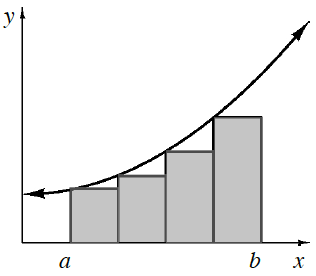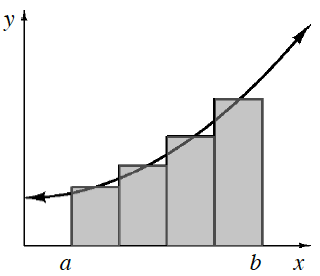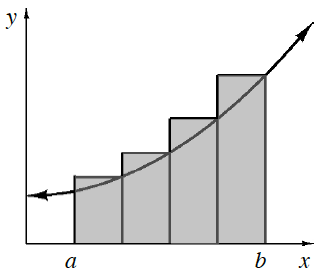### Home > APCALC > Chapter 1 > Lesson 1.3.1 > Problem1-109

1-109.

WHICH IS BETTER? Part One

Below are different sets of rectangles to approximate the area under a curve for the same interval. Look at the three different sets of rectangles and decide which will best approximate the area under the curve of this function for $a ≤ x ≤ b$.

1. Explain why your choice will determine the best approximation for the area.

Which graph is the closest to the exact area under the curve?

Midpoint Rectangles

2. Will left endpoint rectangles always be an underestimate for any function? Explain.

Notice that the graphs in the diagrams above show an increasing function. That is, as $x$ increases, the $y$-values increase. What if we graphed a decreasing function instead? Would left-endpoint rectangles be underestimates then?Left Endpoint RectanglesMidpoint RectanglesRight Endpoint Rectangles